# 1 python大数据挖掘系列之基础知识入门

#### preface

Python在大数据行业非常火爆近两年，as a pythonic，所以也得涉足下大数据分析，下面就聊聊它们。

#### 预先善其事必先利其器

1. numpy 高效处理数据，提供数组支持，很多模块都依赖它，比如pandas，scipy，matplotlib都依赖他，所以这个模块都是基础。所以必须先安装numpy。
2. pandas 主要用于进行数据的采集与分析
3. scipy 主要进行数值计算。同时支持矩阵运算，并提供了很多高等数据处理功能，比如积分，微分方程求样等。
4. matplotlib 作图模块，结合其他数据分析模块，解决可视化问题
5. statsmodels 这个模块主要用于统计分析
6. Gensim 这个模块主要用于文本挖掘
7. sklearn，keras 前者机器学习，后者深度学习。

##### numpy模块安装与使用

###### numpy简单使用
``````import numpy

x=numpy.array([11,22,33,4,5,6,7,])  #创建一维数组
x2=numpy.array([['asfas','asdfsdf','dfdf',11],['1iojasd','123',989012],["jhyfsdaeku","jhgsda"]])    #创建二维数组,注意是([])

x.sort()   #排序，没有返回值的，修改原处的值，这里等于修改了X
x.max()    # 最大值，对二维数组都管用
x.min()    # 最小值，对二维数组都管用
x1=x[1:3]   # 取区间，和python的列表没有区别``````
###### 生成随机数

``````#numpy.random.random_integers(最小值,最大值,个数)  获取的是正数
data = numpy.random.random_integers(1,20000,30)   #生成整形随机数
#正态随机数  numpy.random.normal(均值,偏离值,个数)  偏离值决定了每个数之间的差 ,当偏离值大于开始值的时候，那么会产生负数的。
data1 = numpy.random.normal(3.2,29.2,10)    # 生成浮点型且是正负数的随机数``````

#### pandas

``````print(b)
0     1     2    3
0     1     2     3  4.0
1  sdaf  dsaf  18hd  NaN
2  1463  None  None  NaN``````

``````import pandas
a=pandas.Series([1,2,3,34,])   # 等于一维数组
b=pandas.DataFrame([[1,2,3,4,],["sdaf","dsaf","18hd"],])   # 二维数组
print(b.tail())   # 默认取尾部前后5行
print(b.tail(1))     # 直接传入参数，如我写的那样``````

``````# print(b.describe())   # 显示统计数据信息
3      # 3表示这个二维数组总共多少个元素
count  1.0      # 总数
mean   4.0      # 平均数
std    NaN      # 标准数
min    4.0      # 最小数
25%    4.0      # 分位数
50%    4.0      # 分位数
75%    4.0      # 分位数
max    4.0      # 最大值``````

``````print(b.T)   # 转置
0     1     2
0  1  sdaf  1463
1  2  dsaf  None
2  3  18hd  None
3  4   NaN   NaN``````
##### 通过pandas导入数据

pandas支持多种输入格式，我这里就简单罗列日常生活最常用的几种，对于更多的输入方式可以查看源码后者官网。

##### CSV文件

csv文件导入后显示输出的话，是按照csv文件默认的行输出的，有多少列就输出多少列，比如我有五列数据，那么它就在prinit输出结果的时候，就显示五列

``````csv_data = pandas.read_csv('F:\Learnning\CSDN-python大数据\hexun.csv')
print(csv_data)``````
###### excel表格

``````excel_data = pandas.read_excel('F:\Learnning\CSDN-python大数据\cxla.xls')
print(excel_data)``````
###### 读取SQL

``````conn=pymysql.connect(host="127.0.0.1",user="root",passwd="root",db="test")
sql="select * from fortest"
###### 读取HTML

``````html_data = pandas.read_html('F:\Learnning\CSDN-python大数据\shitman.html')   # 读取本地html文件。
html_from_online = pandas.read_html('https://book.douban.com/')  # 读取互联网的html文件
print(html_data)
print('html_from_online')``````

###### 读取txt文件

``````text_data = pandas.read_table('F:\Learnning\CSDN-python大数据\dforsay.txt')
print(text_data)``````

#### matplotlib 数据可视化分析

``````from matplotlib import  pylab
import numpy
# 下面2行定义X轴，Y轴
x=[1,2,3,4,8]
y=[1,2,3,4,8]
# plot的方法是这样使用（x轴数据,y轴数据,展现形式）
pylab.plot(x,y)   # 先把x,y轴的信息塞入pylab里面，再调用show方法来画图
pylab.show()    # 这一步开始画图,默认是至线图``````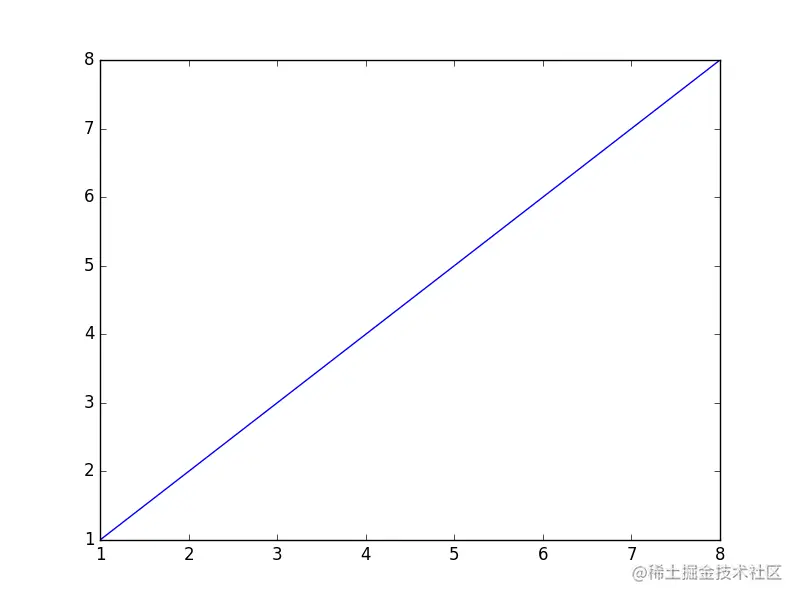#### 下面说说修改图的样式

###### 关于图形类型，有下面几种：
1. 直线图（默认）
2. `-` 直线
3. -- 虚线
4. -. -.形式
5. : 细小虚线
1. c-青色
2. r-红色
3. m-品红
4. g-绿色
5. b-蓝色
6. y-黄色
7. k-黑色
8. w-白色
###### 关于形状，有下面几种：
1. s 方形
2. `*` 星形
3. p 五角形

``````pylab.plot(x,y,'or')   # 添加O表示画散点图，r表示red
pylab.show()``````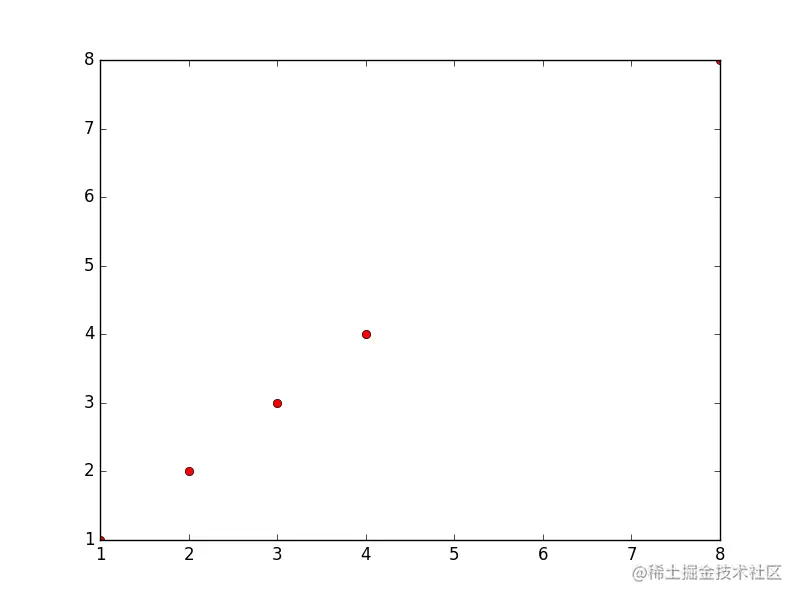``````pylab.plot(x,y,'r:')
pylab.show()``````

``````pylab.plot(x,y,'pr--')   #p是图形为五角星，r为红色，--表示虚线
pylab.title('for learnning')   # 图形标题
pylab.xlabel('args')   # x轴标签
pylab.ylabel('salary')   # y轴标签
pylab.xlim(2)    # 从y轴的2开始做线
pylab.show()``````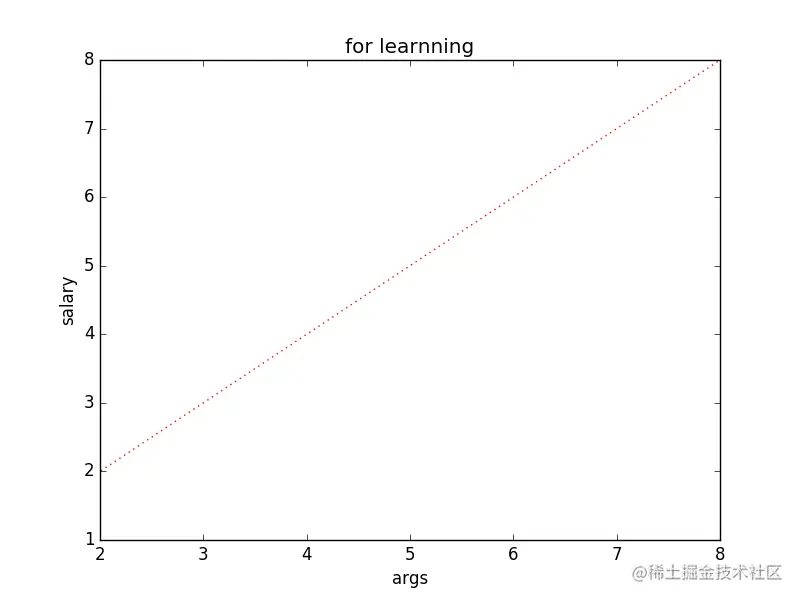##### 直方图

``````data1 = numpy.random.normal(5.0,4.0,10)   # 正态随机数
pylab.hist(data1)
pylab.show()``````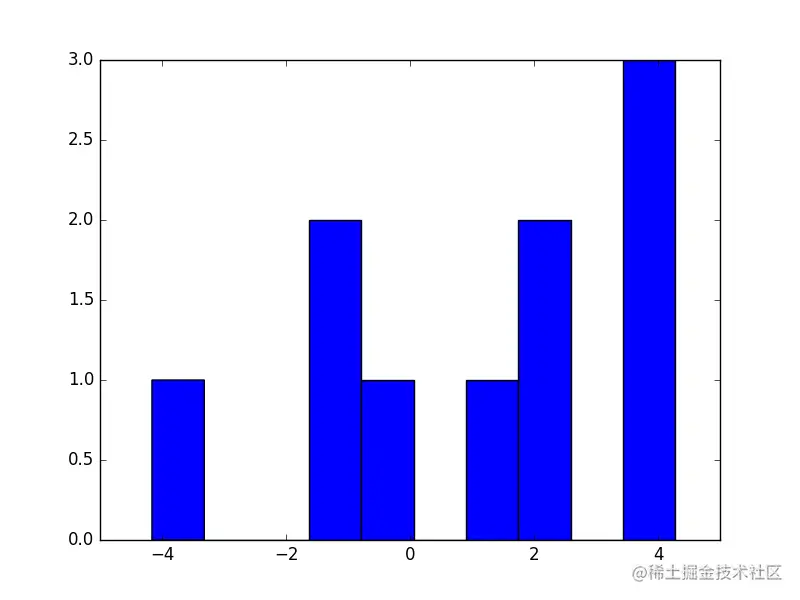Y轴为出现的次数，X轴为这个数的值（或者是范围）

###### 还可以指定直方图类型通过histtype参数：

1. bar ：is a traditional bar-type histogram. If multiple data
are given the bars are aranged side by side.
2. barstacked ：is a bar-type histogram where multiple
data are stacked on top of each other.
3. step ：generates a lineplot that is by default
unfilled.
4. stepfilled ：generates a lineplot that is by default
filled.

``````sty=numpy.arange(1,30,2)
pylab.hist(data1,histtype='stepfilled')
pylab.show() ``````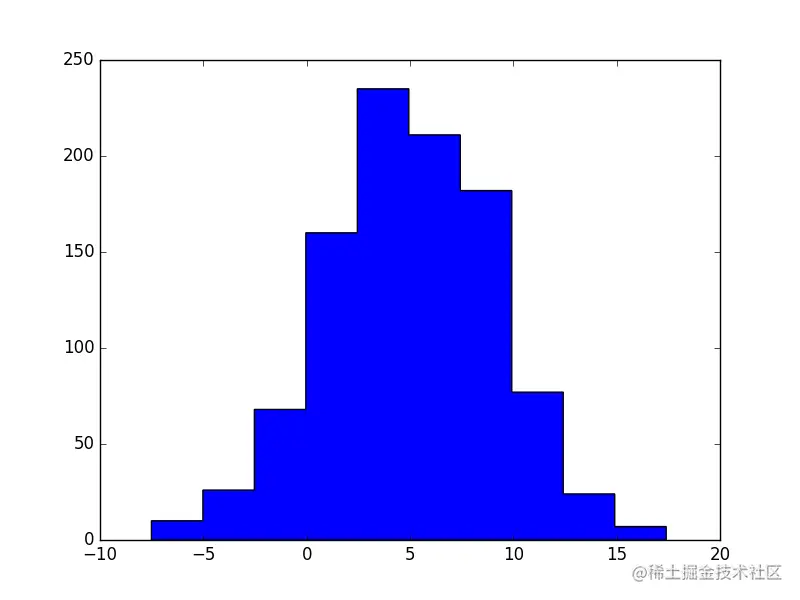##### 子图功能

``````#subplot(行,列,当前区域)
pylab.subplot(2,2,1)   # 申明一个大图里面划分成4块（即2*2），子图使用第一个区域（坐标为x=1,y=1）
pylab.subplot(2,2,2)  # 申明一个大图里面划分成4块（即2*2），子图使用第二个区域（坐标为x=2,y=2）
x1=[1,4,6,9]
x2=[3,21,33,43]
pylab.plot(x1,x2)   # 这个plot表示把x,y轴数据塞入前一个子图中。我们可以在每一个子图后使用plot来塞入x,y轴的数据
pylab.subplot(2,1,2)  # 申明一个大图里面划分成2块（即），子图使用第二个区域（坐标为x=1,y=2）
pylab.show()``````### 实践小例子

``````
dt = csv_data.T   # 装置下，把阅读数和评论数转为行
pylab.show()``````